## Definition Of SAS Congruency Postulate

SAS postulate states, "If two sides and the included angle of one triangle are congruent to two sides and the included angle of another triangle, then the two triangles are congruent."

### More About SAS Congruency Postulate

The angle between any two sides of a triangle is called the included angle of the triangle.

### Example of SAS Congruency Postulate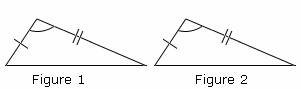In the figures shown, it is clear that two sides and one angle of these triangles are same. So, by SAS postulate these two triangles are congruent.

### Solved Example on SAS Congruency Postulate

#### Ques: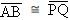and m∠B = m∠Q. What additional information is required to prove that ΔABC ≅ ΔPQR by the SAS postulate?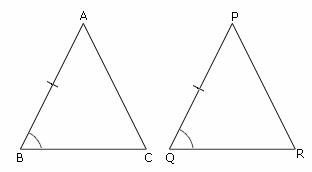##### Choices: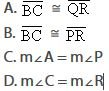Step 2: Hence,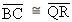, then by the SAS postulate, ΔABC ≅ ΔPQR.